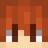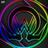This is a lesson on how to make a custom expression. We'll be making an expression for `[the] server's name`

Requirements:
skript-reflect or skript-mirror
skript

Expression:

``````[the] server's name
``````

First, you want to use the custom expression event.

``````expression [the] server's name:
``````

If you want to use multiple patterns, use the patterns section.

``````expression:
patterns:
[the] server's name
name of [the] server
``````

If you want to use the expression you'll need to use the get expression.

``````expression:
patterns:
[the] server's name
name of [the] server
get:
return "name"
``````

We can use a variable to store this value and use `change value` to get the value that you want to change to.

``````expression:
patterns:
[the] server's name
name of [the] server
get:
return {_name}
set:
set {_name} to change value
``````

We can also specify a return type which will make it easier to debug if the incorrect type is given.

``````expression:
patterns:
[the] server's name
name of [the] server
return type: string
get:
return {_name}
set:
set {_name} to change value
``````

We can additionally add a parse section which will execute once it has been parsed. You may use the continue statement if it was successful or the exit statement if there is an error.

``````expression:
patterns:
[the] server's name
name of [the] server
return type: string
parse:
# optional
get:
return {_name}
set:
set {_name} to change value
``````

We can also use expressions inside of our expression. We'll create a `%number% multiplied by %number%` expression. We use the expression `expression-%number%` to get the value of an expression. In this example we use `expression-1` and `expression-2` to get the first and second number.

``````expression %number% multiplied by %number%:
return type: number
get:
return expression-1 * expression-2
``````

Add a comment if you have any questions.

##### You must be logged in to comment

•###### March 19, 2022, 7:40 a.m. - BdawgTheSlaya  ¶

+1

Great tutorial :D

|
•###### Sept. 3, 2022, 10:14 a.m. - ANDREI123  ¶

It's a good tutorial. I would recommend this method to anyone wanting to make their own Expressions.

|
•###### Oct. 14, 2022, 5:20 p.m. - iArx  ¶

Very interesting learned something new

|